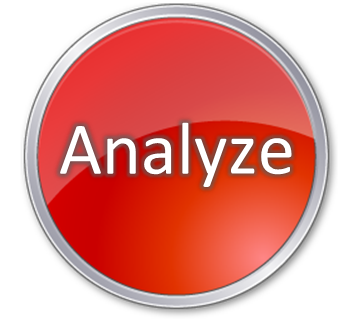Not a member yet?Phoenix Checklist

The Problem

• Why is it necessary to solve the problem?
• What benefits would be received by solving the problem?
• What is the unknown?
• What is it you don’t yet understand?
• What is the information you have?
• What isn’t the problem?
• Is the information sufficient? Or is it insufficient? Or redundant? Or contradictory?
• Where are the boundaries of the problem? Can you separate the various parts of the problem? Can you write them down? What are the relationships of the parts of the problem?
• What are the constants (things that can’t be changed) of the problem?
• Have you seen this problem before?
• Have you seen this problem in a slightly different form?
• Do you know a related problem?
• Try to think of a familiar problem having the same or similar unknown?
• Suppose you find a problem related to yours that has already been solved. Can you use it? Can you use its method?
• Can you restate your problem? How many different ways can you restate it? More general? More specific?
• Can the rules be changed?
• What are the best, worst, and most probable cases you can imagine?

The Plan

• Can you solve the whole problem? Part of the problem?
• What would you like the resolution to be? Can you picture it?
• How much of the unknown can you determine?
• Can you derive something useful from the information you have?
• Have you used all the information?
• Have you taken into account all essential notions in the problem?
• Can you separate the steps in the problem-solving process? Can you determine the correctness of each step?
• What creative thinking techniques can you use to generate ideas? How many different techniques?
• Can you see the result? How many different kinds of results can you see?
• How many different ways have you tried to solve the problem?
• Can you intuit the problem? Can you check the result?
• What should be done? How should it be done?
• Where should it be done?
• When should it be done?
• Who should do it?
• What do you need to do at this time?
• Who will be responsible for what?
• Can you use this problem to solve some other problem?
• What is the unique set of qualities that makes this problem different from another?
• What milestones can best mark your progress?
• How will you know when you are successful?Source:

Thinkertoys: A Handbook of Creative-Thinking Techniques, Michael Michalko, Berkeley, CA: Ten Speed Press, 1991.